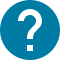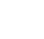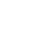学部前期課程
HOME 学部前期課程 数学Ⅰ②(PEAK)

# 数学Ⅰ②(PEAK)

Multivariable calculus
This course covers the differentiation and integration of a function of several variables. It mainly consists of three parts: partial derivatives, multiple integrals and vector calculus. Improper integral for single variable function, implicit function theorem will also be taught. Some applications of these mathematical concepts will be introduced. This course is a continuation of the single-variable Calculus that I taught in Autumn of 2020.

コース名

30960
CAS-PF1712L3

S1 S2

2

NO

30961
CAS-PF1712L3

S1 S2

2

NO

I will upload each week's materials to ITC-LMS. You can also contact me by sending e-mails if you have any questions. This semester we plan to study the following topics. 1 partial derivatives 2 tangent plane and chain rule 3 Taylor's theorem and extreme values 4 implicit function theorem 5 double integral and integration methods 6 change of variables in double integrals 7 improper integral 8 some concepts in vector calculus (if time permits)

To be determined. Lecture notes will be distributed each week.

To be determined.

Students who take the course are required to have the knowledge of single-variable calculus.